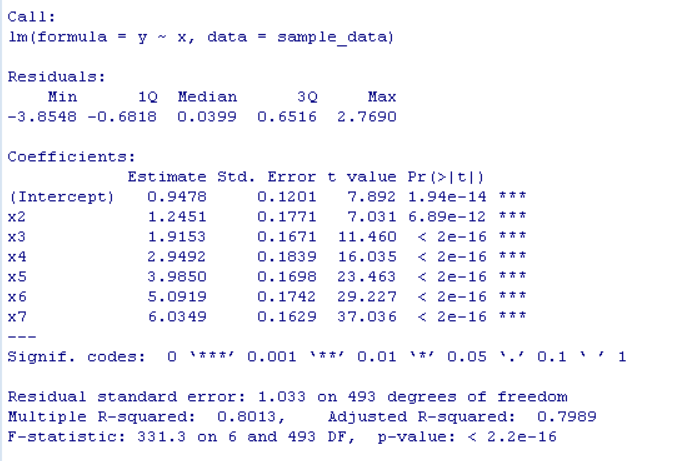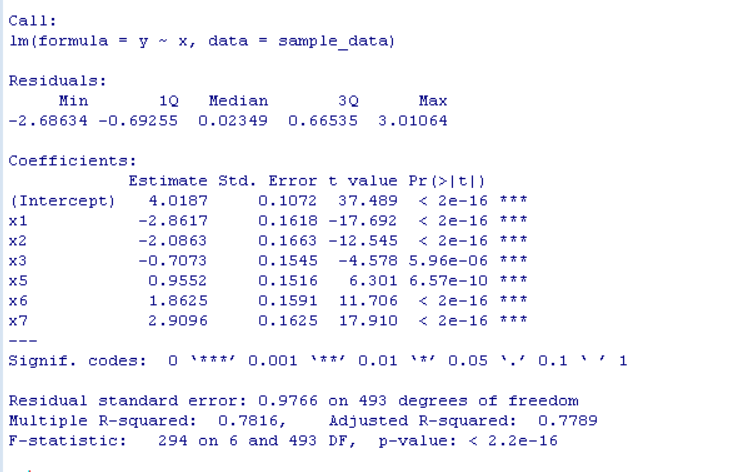Open in App
Not now

# Specify Reference Factor Level in Linear Regression in R

• Last Updated : 23 Feb, 2022

In this article, we will discuss how to specify Reference Factor Level in Linear Regression in the R Programming Language.

In a basic linear regression model in the R Language, by default, the reference category 1 is used for the factor variable. But sometimes we need to manually set the reference factor level in the linear regression model. To do so we use the relevel() function of the R Language. The relevel() function is used to reorder levels of a factor vector. The levels of a factor vector are re-ordered so that the level specified by the user is first and the others are moved down one step.

## Linear regression model with default reference factor level

To create a basic linear regression model, we use the lm() function of the R Language. The lm() function is used to fit linear models to a data frame in the R Language. It can be used to carry out linear regression for the prediction of unknown data. The lm() function takes the data frame and fitting function as the argument and returns a trained linear regression model.

Syntax:

lm( fitting_function, data )

Parameter:

• fitting_function: determines the function used to fit the data frame.
• data: determines the data frame used in linear regression.

Example:

Here is a basic linear regression model with the default reference factor level.

## R

 `# create sample data frame``x <- ``sample``(1:7, 500, replace = ``TRUE``)``y <- ``round``(x + ``rnorm``(500), 3)``x <- ``as.factor``(x)``sample_data <- ``data.frame``(x, y)` `# create linear model``linear_model <- ``lm``( y~x, sample_data)` `# print summary of linear model``summary``(linear_model)`

Output:

Here, by default the factor reference level is x1.## Linear regression model with manual reference factor level

To specify the manual reference factor level in the R Language, we will use the relevel() function. The relevel() function is used to reorder the factor vector so that the level specified by the user is first and others are moved down. The relevel() function takes factor vector and reference level as argument and returns the factor vector with levels reordered according to the reference level set by the user.

Syntax:

relevel( factor_vector, ref )

Parameter:

• factor_vector: determines the factor vector which is to be reordered.
• ref: determines the reference level according to which factor is to be reordered.

Example:

Here is a basic linear regression model with the factor reference level set to 4.

## R

 `# create sample data frame``x <- ``sample``(1:7, 500, replace = ``TRUE``)``y <- ``round``(x + ``rnorm``(500), 3)``x <- ``as.factor``(x)``sample_data <- ``data.frame``(x, y)` `# refactor reference level``sample_data\$x <- ``relevel``(sample_data\$x, ref = 4)` `# create linear model``linear_model <- ``lm``( y~x, sample_data)` `# print summary of linear model``summary``(linear_model)`

Output:My Personal Notes arrow_drop_up